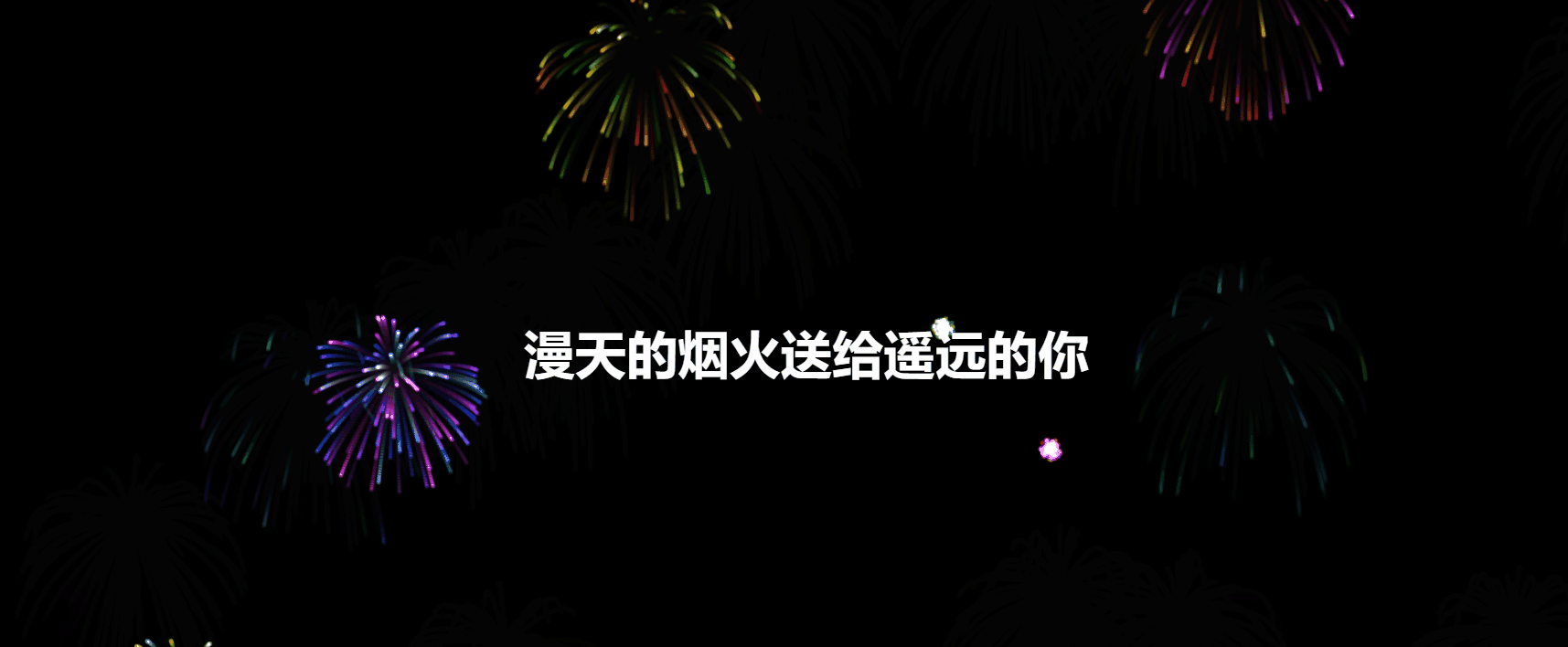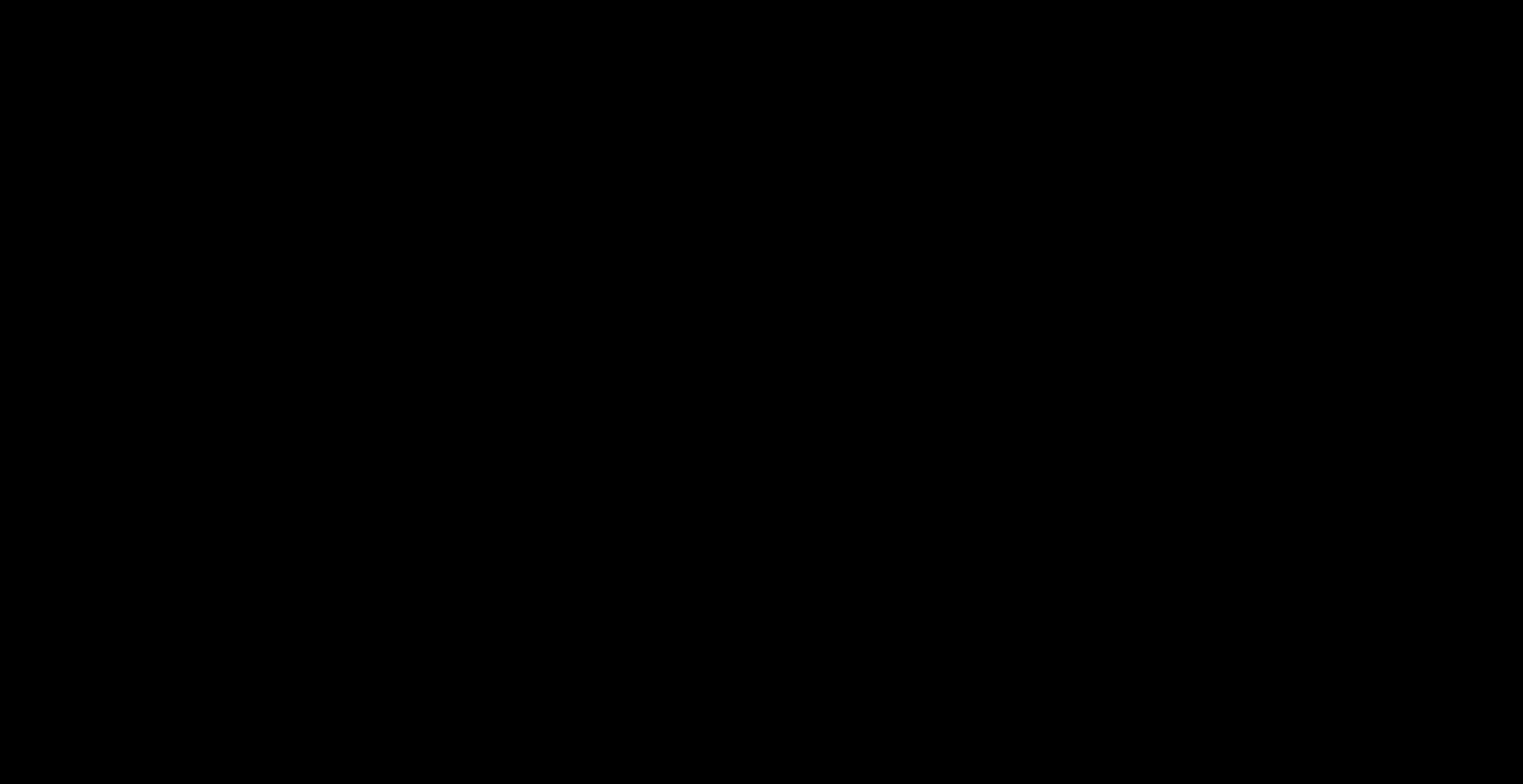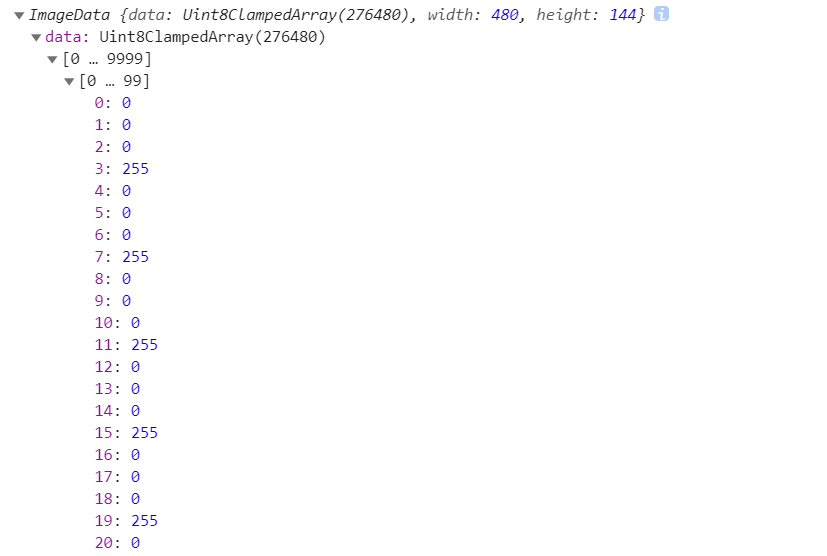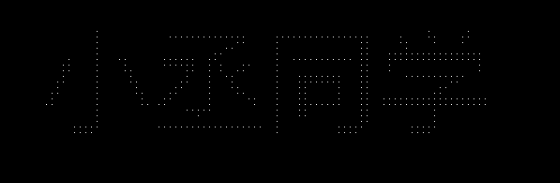# 还在用canvas画格子吗？文字烟花效果更不错噢

💞“在人间贩卖声音 等凑够满天星辰 放烟花给你看## 实现效果## 实现过程

### 1. 在画布上绘制文字

1. `ctx.font`至少需要两个参数，一个字体大小，一个字体
2. 这里的颜色之所以设为`#000001`原因是背景是黑色的，这样这个字不会被看到，但是它是真实存在的，不然每次点击时都会有一个字体生成在左上角，影响视觉，当然也可以新建在一个画布，这里就简单处理了
3. `ctx.measureText(text)`：返回一个对象，该对象包含以像素计的指定字体宽度
``````// 填充字体样式
let font = 120
ctx.font = font + "px '微软雅黑'"
ctx.fillStyle = "#000001"
// 内容
let text = '小丞同学'
// 获取字体的宽度
let textWidth = ctx.measureText(text).width
// 在左上角填充字体
ctx.fillText(text,0 , font)

### 2. 获取像素点

• R - 红色 (0-255)
• G - 绿色 (0-255)
• B - 蓝色 (0-255)
• A - alpha 通道 (0-255; 0 是透明的，255 是完全可见的)

``````let imgData = ctx.getImageData(0, 0, textWidth, font * 1.2)### 3. 实现文字粒子化

``````for (let h = 0; h < font * 1.2; h += 6) {
for (let w = 0; w < textWidth; w += 6) {
let position = (textWidth * h + w) * 4;
// 返回的数组是rgba的方式存储
let r = imgData.data[position],
g = imgData.data[position + 1],
b = imgData.data[position + 2],
a = imgData.data[position + 3];
if (r + g + b == 0) {
continue
}
//烟花代码
}
}``````let firework = {};
firework.x = x;
firework.y = y;
firework.fx = x + w - textWidth / 2;
firework.fy = y + h - font / 2;
firework.size = Math.floor(Math.random() * 2) + 1;
firework.speed = 1;
setColors(firework);
fireworks.push(firework);

### 4. 设置粒子动画

``````firework.x += (firework.fx - firework.x) / 10;
firework.y += (firework.fy - firework.y) / 10 - (firework.alpha - 1) * firework.speed;
firework.alpha -= 0.01;
// 如果透明度小于0就删除这个粒子
if (firework.alpha <= 0) {
fireworks.splice(i, 1);
// 跳过这次循环，不进行绘制
continue;
}

### 5. 设置拖尾并渲染更新画布

``````function tick() {
// // 设置拖影
ctx.globalCompositeOperation = 'destination-out';
ctx.fillStyle = 'rgba(0,0,0,' + 10 / 100 + ')';
ctx.fillRect(0, 0, canvas.width, canvas.height);
ctx.globalCompositeOperation = 'lighter';
// 更新画布
drawFires();
requestAnimationFrame(tick);
}

## 总结